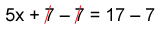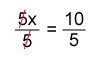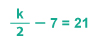# How to Solve Two-Step Equations

1. Math Lessons >
2. Two-step Equations

What is a two-step equation?

As the name suggests, a two-step equation requires two steps to solve a problem. A two-step equation has more than one operation.

An example of two-step equation is

9k + 3 = 21

How do you solve a two-step equation?

It doesn't take much to solve a two-step equation. Isolate the variable to solve the equation. To isolate the variable, use inverse operations wherever required.

Let's consider the below instance:

5x + 7 = 17

Step 1: In the above-given equation, undo the addition using inverse operations (subtraction) on both sides of the equation.5x = 10

Step 2: To remove the coefficient of the variable, perform the inverse of multiplication (division). Divide by 5 on both sides of the equation.x = 2

Plug-in the value of the variable in the equation to check your answer.

For the equation 5x + 7 = 17, substitute x = 2,

(5 × 2) + 7 = 17

10 + 7 = 17

17 = 17

The lesson in a nutshell:

To obtain the value of a variable, we have to isolate the variable.

Perform inverse operations on both sides of the equation to isolate the variable.

Simplify the equation and find the value of the variable.Boost your skills using our free printable worksheets on Two-step Equations.

Solved Examples:

Example 1

1. Solve 4x – 7 = 15

4x – 7 = 15

4x – 7 + 7 = 15 + 7

[ Adding 7 on both sides ]

4x = 22

4x4 = 224

[ Dividing by 4 on both sides ]

x = 112

Example 2

2. Solve 8y = 40 – 2y

8y = 40 – 2y

8y + 2y = 40 – 2y + 2y

[ Adding 2y on both sides ]

10y = 40

10y10 = 4010

[ Dividing by 10 on both sides ]

y = 4

Example 3

3. On a clearance sale, a jumpsuit was up for grabs at half the price. Sara grabs the deal, and she further uses a coupon for \$7. If she pays \$21 to the cashier, what was the original cost of the jumpsuit?

The above scenario forms an equation:, where k refers to the original cost of the jumpsuit.

k2 – 7 = 21

k2 – 7 + 7 = 21 + 7

[ Adding 7 on both sides ]

k2 = 28

k2 × 2 = 28 × 2

[ Multiplying 2 on both sides ]

k = 56

The original cost of the jumpsuit is \$56.